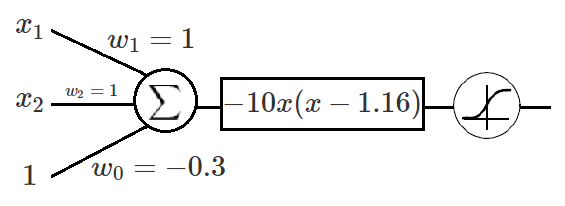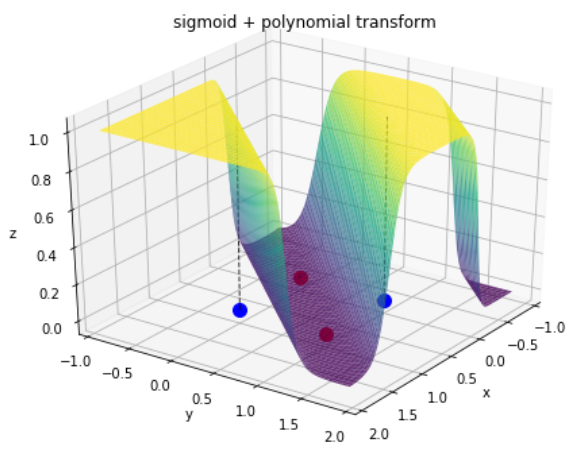# Solving XOR with a single Perceptron

#### Advocating for polynomial transformations as a way to increase the representational power of artificial neurons.

1 – Introduction

Everyone who has ever studied about neural networks has probably already read that a single perceptron can’t represent the boolean XOR function. The book Artificial Intelligence: A Modern Approach, the leading textbook in AI, says: “[XOR] is not linearly separable so the perceptron cannot learn it” (p.730). Wikipedia agrees by stating: “Single layer perceptrons are only capable of learning linearly separable patterns”. The Deep Learning book, one of the biggest references in deep neural networks, uses a 2 layered network of perceptrons to learn the XOR function so the first layer can “ learn a different [linearly separable] feature space” (p.168).

Since its creation, the perceptron model went through significant modifications. We discovered different activation functions, learning rules and even weight initialization methods. Because of these modifications and the development of computational power, we were able to develop deep neural nets capable of learning non-linear problems significantly more complex than the XOR function. The only caveat with these networks is that their fundamental unit is still a linear classifier. So their representational power comes from their multi-layered structure, their architecture and their size.

Trying to improve on that, I’d like to propose an adaptive polynomial transformation in order to increase the representational power of a single artificial neuron. In the next section I’ll quickly describe the original concept of a perceptron and why it wasn’t able to fit the XOR function. I’ll then overview the changes to the perceptron model that were crucial to the development of neural networks. In section 4, I’ll introduce the polynomial transformation and compare it to the linear one while solving logic gates. Finally I’ll comment on what I believe this work demonstrates and how I think future work can explore it.

2 – The Perceptron and its Nemesis in the 60s

The perceptron is a model of a hypothetical nervous system originally proposed by Frank Rosenblatt in 1958. It was heavily based on previous works from McCullock, Pitts and Hebb, and it can be represented by the schematic shown in the figure below.

As we can see, it calculates a weighted sum of its inputs and thresholds it with a step function. Geometrically, this means the perceptron can separate its input space with a hyperplane. That’s where the notion that a perceptron can only separate linearly separable problems came from. Since the XOR function is not linearly separable, it really is impossible for a single hyperplane to separate it.

An obvious solution was to stack multiple perceptrons together. Although, there was a problem with that. When Rosenblatt introduced the perceptron, he also introduced the perceptron learning rule(the algorithm used to calculate the correct weights for a perceptron automatically). The rule didn’t generalize well for multi-layered networks of perceptrons, thus making the training process of these machines a lot more complex and, most of the time, an unknown process. This limitation ended up being responsible for a huge disinterest and lack of funding of neural networks research for more than 10 years [reference].

3 – The 80s to the rescue!

In 1986, a paper entitled Learning representations by back-propagating errors by David Rumelhart and Geoffrey Hinton changed the history of neural networks research. It introduced a ground-breaking learning procedure: the backpropagation algorithm. The paper proposed the usage of a differentiable function instead of the step function as the activation for the perceptron. With this modification, a multi-layered network of perceptrons would become differentiable. Hence gradient descent could be applied to minimize the network’s error and the chain rule could “back-propagate” proper error derivatives to update the weights from every layer of the network.

Fast forward to today and we have the most used model of a modern perceptron a.k.a. an artificial neuron. Even though it doesn’t look much different, it was only on 2012 that Alex Krizhevsky was able to train a big network of artificial neurons that changed the field of computer vision and started a new era in neural networks research.

The only noticeable difference from Rosenblatt’s model to the one above is the differentiability of the activation function. Since 1986, a lot of different activation functions have been proposed. See some of the most popular examples below.

Each one of these activation functions has been successfully applied in a deep neural network application and yet none of them changed the fact that a single neuron is still a linear classifier.

4 – Hyperplanes… Hyperplanes everywhere

Let’s go back to logic gates. Take a look at a possible solution for the OR gate with a single linear neuron using a sigmoid activation function.

The learned hyperplane is determined by equation 1. The equation is factored into two parts: a constant factor, that impacts directly on the sharpness of the sigmoidal curve; and the equation to a hyperplane that separates the neuron’s input space.

Now, let’s take a look at a possible solution for the XOR gate with a 2 layered network of linear neurons using sigmoid functions as well.

The hyperplanes learned by each neuron are determined by equations 2, 3 and 4. Just like in equation 1, we can factor the following equations into a constant factor and a hyperplane equation. What is interesting, though, is the fact that the learned hyperplanes from the hidden layers are approximately parallel.

From the approximations demonstrated on equations 2 and 3, it is reasonable to propose a quadratic polynomial that has the two hyperplanes from the hidden layers as its roots (equation 5). By refactoring this polynomial (equation 6), we get an interesting insight.

From equation 6, it’s possible to realize that there’s a quadratic polynomial transformation that can be applied to a linear relationship between the XOR inputs and result in two parallel hyperplanes splitting the input space. Therefore, it’s possible to create a single perceptron, with a model described in the following figure, that is capable of representing a XOR gate on its own.Perceptron’s schematic modified with polynomial learned from the 2-layered network XOR representation.

As in equations 1, 2 and 3, I included a constant factor to the polynomial in order to sharpen the shape of the resulting sigmoidal curves. The negative sign came from the sign of the multiplication of the constants in equations 2 and 3. We can see the result in the following figure.

Now, let’s modify the perceptron’s model to introduce the quadratic transformation shown before. In order to avoid redundant parameters in the linear and the polynomial part of the model, we can set one of the polynomial’s roots to 0. Then, the weights from the linear part of the model will control the direction and position of the hyperplanes and the weights from the polynomial part will control the relative distances between them.

From the model, we can deduce equations 7 and 8 for the partial derivatives to be calculated during the backpropagation phase of training.

After initializing the linear and the polynomial weights randomly (from a normal distribution with zero mean and small variance), I ran gradient descent a few times on this model and got the results shown in the next two figures.

There it is! A single artificial neuron just automatically learned a perfect representation for a non-linear function. It’s interesting to see that the neuron learned both possible solutions for the XOR function, depending on the initialization of its parameters.

Without any loss of generality, we can change the quadratic polynomial in the aforementioned model for an n-degree polynomial. Similar to what we did before to avoid redundancy in the parameters, we can always set one of the polynomial’s roots to 0. The general model is shown in the following figure.

The equations for p(x), its vectorized form and its partial derivatives are demonstrated in 9, 10, 11 e 12.

Let’s see how a cubic polynomial solves the XOR problem.Automatically learned representation for XOR from a single neuron with a cubic transformation.

The bigger the polynomial degree, the greater the number of splits of the input space. Nevertheless, just like with the linear weights, the polynomial parameters can (and probably should) be regularized. Hence an n-degree polynomial is able to learn up to n+1 splits in its input space, depending on the number of real roots it has.

It’s important to remember that these splits are necessarily parallel, so a single perceptron still isn’t able to learn any non-linearity. That’s when the structure, architecture and size of a network comes back to save the day. The goal of the polynomial function is to increase the representational power of deep neural networks, not to substitute them.

5 – So what?

So polynomial transformations help boost the representational power of a single perceptron, but there’s still a lot of unanswered questions. Can they improve deep networks with dozens of layers? How much do they improve and is it worth it? Do they matter for complex architectures like CNNs and RNNs? How big of a polynomial degree is too big? How should we initialize the weights? Which activation function works best with it? And the list goes on.

The good thing is that the linear solution is a subset of the polynomial one. So a polynomial might create more local minima and make it harder to train the network since it’s not monotonic. Nonetheless, if there’s a solution with linear neurons, there’s at least the same solution with polynomial neurons. This could give us some intuition on how to initialize the polynomial weights and how to regularize them properly.

Another great property of the polynomial transformation is that it is computationally cheaper than its equivalent network of linear neurons. In a quadratic transformation, for example, you get a non-linearity per neuron with: only two extra parameters instead of three times the size of your neuron’s input space; and one less matrix multiplication. Depending on the size of your network, these savings can really add up.

I started experimenting with polynomial neurons on the MNIST data set, but I’ll leave my findings to a future article. For now, I hope I was able to get you intrigued about the possibility of using polynomial perceptrons and how to demonstrate they are either great or useless compared to linear ones.Source: Deep Learning on Medium